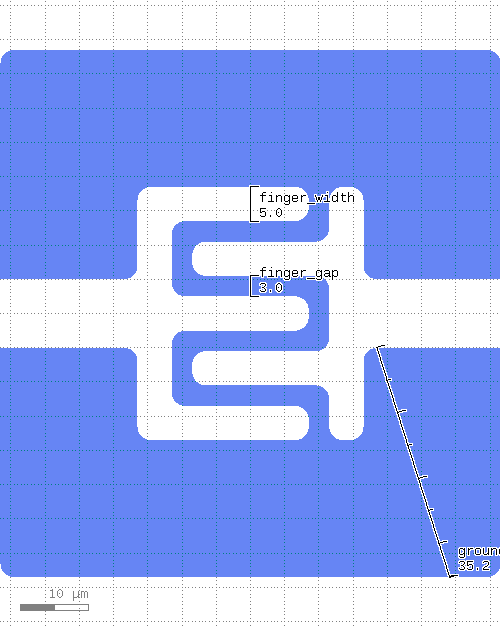# kqcircuits.elements.finger_capacitor_square¶

class kqcircuits.elements.finger_capacitor_square.FingerCapacitorSquare[source]

The PCell declaration for a square finger capacitor.

Two ports with reference points. The arm leading to the finger has the same width as fingers. The feedline has the same length as the width of the ground gap around the coupler.

can_create_from_shape_impl()[source]

default implementation

build()[source]

Child classes re-define this method to build the PCell.

get_ground_region()[source]

Returns the ground region for the finger capacitor.

finger_area_width()[source]
finger_area_length()[source]
cut_region(region, x_max, y_max)[source]
classmethod get_sim_ports(simulation)[source]

List of RefpointToSimPort objects defining which refpoints should be turned to simulation ports for the given element class

Returns empty list if not implemented for Element subclass. When implementing this method, the best practice is for this method to have no “side effects”, that is all code contained within this method should only serve to derive the list of RefpointToSimPort objects and nothing else: no change in element’s geometry or parameter values.

Parameters
• cls – Element class, this is a class method

• simulation – Simulation object where a cell of this element class is placed. Use this argument if you need to decide certain arguments for RefpointToSimPort objects based on simulation’s parameters

Returns

List of RefpointToSimPort objects, empty list by default

PCell parameters:

• a2 (Double) - Non-physical value ‘-1’ means that the default size ‘a’ is used., default=`-1`, unit=`μm`

• b2 (Double) - Non-physical value ‘-1’ means that the default size ‘b’ is used., default=`-1`, unit=`μm`

• finger_gap_end (Double) - Gap between the finger and other pad, default=`3`, unit=`μm`

• ground_padding (Double) - Ground plane padding, default=`20`, unit=`μm`

• fixed_length (Double) - Fixed length of element, 0 for auto-length, default=`0`, unit=`μm`

• ground_gap_ratio (Double) - Ground connection width per gap ratio, default=`0`, unit=`μm`

• a (Double) - Width of center conductor, default=`10`, unit=`μm`

• b (Double) - Width of gap, default=`6`, unit=`μm`

• n (Int) - Number of points on turns, default=`64`

• r (Double) - Turn radius, default=`100`, unit=`μm`

• margin (Double) - Margin of the protection layer, default=`5`, unit=`μm`

• face_ids (List) - Chip face IDs list, default=`['1t1', '2b1', '1b1', '2t1']`

• display_name (String) - Name displayed in GUI (empty for default), default=

• protect_opposite_face (Boolean) - Add opposite face protection too, default=`False`

• finger_number (Int) - Number of fingers, default=`5`

• finger_width (Double) - Width of a finger, default=`5`, unit=`μm`

• finger_gap (Double) - Gap between the fingers, default=`3`, unit=`μm`

• finger_length (Double) - Length of the fingers, default=`20`, unit=`μm`

• corner_r (Double) - Corner radius, default=`2`, unit=`μm`